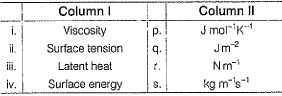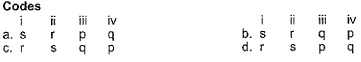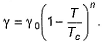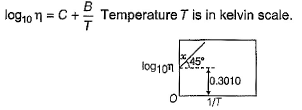NEET  >  Test: Viscosity & Surface Tension

# Test: Viscosity & Surface Tension

Test Description

## 20 Questions MCQ Test Chemistry Class 11 | Test: Viscosity & Surface Tension

Test: Viscosity & Surface Tension for NEET 2023 is part of Chemistry Class 11 preparation. The Test: Viscosity & Surface Tension questions and answers have been prepared according to the NEET exam syllabus.The Test: Viscosity & Surface Tension MCQs are made for NEET 2023 Exam. Find important definitions, questions, notes, meanings, examples, exercises, MCQs and online tests for Test: Viscosity & Surface Tension below.
Solutions of Test: Viscosity & Surface Tension questions in English are available as part of our Chemistry Class 11 for NEET & Test: Viscosity & Surface Tension solutions in Hindi for Chemistry Class 11 course. Download more important topics, notes, lectures and mock test series for NEET Exam by signing up for free. Attempt Test: Viscosity & Surface Tension | 20 questions in 20 minutes | Mock test for NEET preparation | Free important questions MCQ to study Chemistry Class 11 for NEET Exam | Download free PDF with solutions
 1 Crore+ students have signed up on EduRev. Have you?
Test: Viscosity & Surface Tension - Question 1

### Which of the following properties of water can be used to explain the spherical shape of rain droplets?

Detailed Solution for Test: Viscosity & Surface Tension - Question 1

A simple way to form a drop is to allow liquid to flow slowly from the lower end of a vertical tube of small diameter. The surface tension of the liquid causes the liquid to hang from the tube, forming a pendant. When the drop exceeds a certain size it is no longer stable and detaches itself. The falling liquid is also a drop held together by surface tension.

Test: Viscosity & Surface Tension - Question 2

### How does the surface tension of a liquid vary with increase in temperature?

Detailed Solution for Test: Viscosity & Surface Tension - Question 2

In general, surface tension decreases when temperature increases because cohesive forces decrease with an increase of molecular thermal activity. The influence of the surrounding environment is due to the adhesive action liquid molecules have at the interface.

Test: Viscosity & Surface Tension - Question 3

### Arrange the following in increasing order of surface tension. I (water), II (ethanol), III (hexane)

Detailed Solution for Test: Viscosity & Surface Tension - Question 3

The magnitude of surface tension of liquid depends on the attractive forces between the molecules. In ethanol and water, we have hydrogen bonding. However water has more effective H-bonding owing to its small size. Hexane has induced dipole-induced dipole force which is a weak force. So the order is
III<II<I

Test: Viscosity & Surface Tension - Question 4

Some of the following properties of liquids arise because of the molecular interaction and thermal energy.
I. Vapour pressure II. Surface tension III. Viscosity

Select the properties.

Detailed Solution for Test: Viscosity & Surface Tension - Question 4

Vapour pressure depends on both molecular interaction and thermal energy within molecular attraction vapour pressure decreases and with increase in thermal energy vapour pressure increases.
Surface tension depends on both molecular interaction and thermal energy with increase in molecular interaction surface tension increases.
Viscosity is also depends on Molecular interaction, with increase in molecular attraction viscosity increases.

Test: Viscosity & Surface Tension - Question 5

One of the following properties increase with increase in temperature,

Detailed Solution for Test: Viscosity & Surface Tension - Question 5

Vapor pressure or equilibrium vapor pressure is defined as the pressure exerted by a vapor in thermodynamic equilibrium with its condensed phases at a given temperature in a closed system. The equilibrium vapor pressure is an indication of a liquid's evaporation rate.

Test: Viscosity & Surface Tension - Question 6

Which is equal to 1 kg m-1 S-1?

Detailed Solution for Test: Viscosity & Surface Tension - Question 6

The correct answer is option D
The SI unit of viscosity is the pascal second [Pa s].
The most common unit of viscosity is the dyne second per square centimeter[dyne s/cm2],
Which is given the name poise [P] after the French physiologist Jean Poiseuille
We know that 1 Pa = 1Nm-2 and 1N = 1kgms-2
So, from these relations we can get,
1 Pa-s = 1Nm-2s = 1 kgm-2 s-1
Ten poise equal one pascal second [Pa s] making the centipoise [cP] and millipascal second [mPa s] identical.
Hence, 10 poise = 1 kg m-1 s-1

Test: Viscosity & Surface Tension - Question 7

Surface tension of water is 73 dynes cm -1 at 20° C. If surface area is increased by 0.10 m2, work done is

Detailed Solution for Test: Viscosity & Surface Tension - Question 7

Work done = surface tension×Change in area
Surface tension = 73 dyne cm-1 or 73 x 10-3 N/m
Area = 0.10 m2
So, work done = 73×10-3 x 0.10
= 73 x 10-4 J or 73×10-4 x 107 erg
= 7.3×104 erg

Test: Viscosity & Surface Tension - Question 8

The coefficient of viscosity of a species is 2.10x 10-5 Pa-s. This value is also equal to

Test: Viscosity & Surface Tension - Question 9

Direction (Q. Nos. 9) Choice the correct combination of elements and column I and coloumn II  are given as option (a), (b), (c) and (d), out of which ONE option is correct.

Q. Match the physical properties given in Column I with the corresponding units given in Column II and select the correct answer from the codes given below.Detailed Solution for Test: Viscosity & Surface Tension - Question 9

Viscosity = kg m-1 s-1
Surface tension = N m-1
Surface energy = J m-2
For latent heat, we have J kg-1 and not as in p.

*Multiple options can be correct
Test: Viscosity & Surface Tension - Question 10

Direction (Q. Nos. 10-12) This section contains 3 multiple choice questions. Each question has four choices (a), (b), (c) and (d), out of which ONE or  MORE THANT ONE  is correct.

Q. Select correct statement(s).

Detailed Solution for Test: Viscosity & Surface Tension - Question 10

The spherical shape is the shape in which it is most stable due to its surface tension. On earth also it is applicable. A drop of water wants to be in a spherical shape but is affected by other forces, so it becomes distorted. The shape is due to surface tension and not pressure. In space there is no force available to distort the spherical shape.
Adding soaps and detergents that disrupt the intermolecular attractions between adjacent water molecules can reduce the surface tension of water. Because they affect the surface properties of a liquid, soaps, and detergents are called surface-active agents, or surfactants.
Surface tension decreases when the temperature increases because cohesive forces decrease with an increase in molecular thermal activity.

*Multiple options can be correct
Test: Viscosity & Surface Tension - Question 11

Select the correct statement(s).

Detailed Solution for Test: Viscosity & Surface Tension - Question 11

a) True, cohesive forces are intermolecular forces between like molecules and adhesive forces between unlike molecules.
b) True, only due to cohesive force, all the molecules of droplets(which are like molecule) are attracted towards each other to form drop
c) True, if the cohesive force becomes weak as to adhesive forces, then there will be no force to bind water molecules and the drop will collapse and spread into film.
d) True, It's only due to cohesive force that mercury doesn’t wet the glass.

*Multiple options can be correct
Test: Viscosity & Surface Tension - Question 12

The temperature dependence of the surface tension of a liquid is given by the Sugden equation,.

Select correct statement(s).

Test: Viscosity & Surface Tension - Question 13

Direction (Q. Nos. 13 and 18) This sectionis based on statement I and Statement II. Select the correct answer from the code given below

Q.

Statement I : Increase in temperature decreases viscosity of liquid.

Statement II : Increase in temperature and thus kinetic energy can overcome intermolecular forces of attraction.

Detailed Solution for Test: Viscosity & Surface Tension - Question 13

Viscosity of liquids decreases as the temperature rises because at high temperature, molecules have high kinetic energy and can overcome the intermolecular forces to slip past one another between the layers.

Test: Viscosity & Surface Tension - Question 14

Statement I : The order of viscosities o f hexane, water and glycerol is hexane < water < glycerol.

Statement II : Hexane has weakest intermolecular forces and glycerol the 1 strongest.

Detailed Solution for Test: Viscosity & Surface Tension - Question 14

In water and glycerine, the intermolecular force that exists is  Hydrogen bonding.
In the case of Hexane, Dispersion forces / London forces exists.
The order of viscosities of these liquids is hexane < water < glycerine.
Hexane has weakest intermolecular forces and glycerine the strongest. ( three OH groups).
Therefore, hexane has minimum viscosity and glycerine has maximum viscosity.

Test: Viscosity & Surface Tension - Question 15

Statement I : Liquids tend to have maximum number of molecules at their surface.

Statement II : Small liquid drops have spherical shape.

Detailed Solution for Test: Viscosity & Surface Tension - Question 15

Liquids try to reduce their surface tension and also the no of molecules at the surface.So statement I is incorrect.
Statement II is correct. Small liquid drops have spherical shape as they try to reduce their surface tension and their surface area. So for a fixed volume, the sphere has the least surface area.

Test: Viscosity & Surface Tension - Question 16

Statement I : As temperature is increased, less work is required to extend the surface of a liquid therefore surface tension decreases.

Statement II : As the temperature and hence the intensity of molecular motion increases, intermolecular forces become less effective.

Detailed Solution for Test: Viscosity & Surface Tension - Question 16

Both statements are true and statement IIis the correct explanation of statement I. As temperature is increased, less work is required to extend the surface of a liquid therefore surface tension decreases. The reason behind is that with increase in temperature, molecular motion increases and so intermolecular forces become less effective.

Test: Viscosity & Surface Tension - Question 17

Statement I : Capillary action is due to surface tension.

Statement II : Mercury, with its strong cohesive forces and weaker adhesive forces, does not show capillary rise.

Test: Viscosity & Surface Tension - Question 18

Statement I : A certain amount of energy called activation energy (Ea) is required to move into a hole.

Statement II : On increasing temperature, Ea is available and liquid can flow more easily thus, viscosity decreases.

Test: Viscosity & Surface Tension - Question 19

Direction (Q. Nos. 11-13) This section contains a paragraph, wach describing theory, experiments, data etc. three Questions related to paragraph have been given.Each question have only one correct answer among the four given options (a),(b),(c),(d).

Variation of viscosity (q) with temperature T is given byQ. What is viscosity at 100 K?

Detailed Solution for Test: Viscosity & Surface Tension - Question 19

From the graph, we can see that;
log10 ƞ = 0.3010 + 1/T
= 0.3010 + 1/100   (at 100K)   -------------(i)
Given that variation of viscosity(q) = log10ƞ with T
Therefore, log10 ƞ = 0.311
As only for log10 ƞ = 0.311, we will have eqn i correct

Test: Viscosity & Surface Tension - Question 20

Variation of viscosity (q) with temperature T is given byQ. Energy of activation required to move into a hole is

## Chemistry Class 11

204 videos|331 docs|229 tests
 Use Code STAYHOME200 and get INR 200 additional OFF Use Coupon Code
Information about Test: Viscosity & Surface Tension Page
In this test you can find the Exam questions for Test: Viscosity & Surface Tension solved & explained in the simplest way possible. Besides giving Questions and answers for Test: Viscosity & Surface Tension, EduRev gives you an ample number of Online tests for practice

## Chemistry Class 11

204 videos|331 docs|229 tests

### How to Prepare for NEET

Read our guide to prepare for NEET which is created by Toppers & the best Teachers